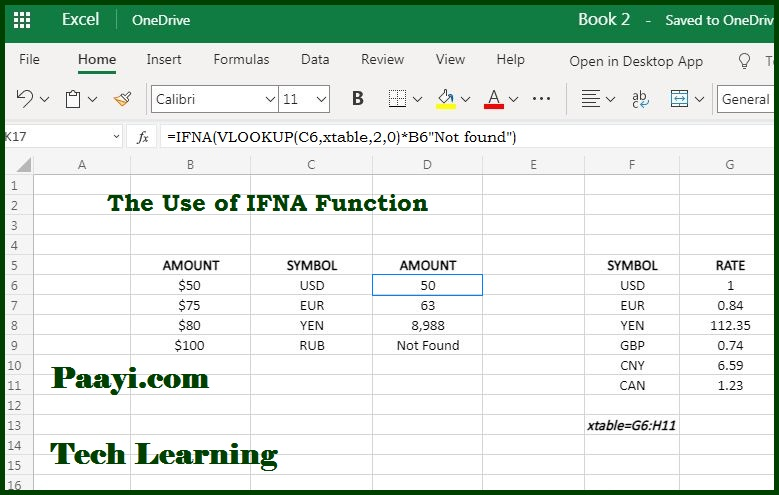# Learn How to Use Microsoft Excel IFNA Function

Written by | 0 Comments | 722 Views

In this article, you will learn how to use the Microsoft Excel IFNA function and its prime function in Microsoft Excel. You will also get to know the Microsoft Excel IFNA function return value and syntax with the help of some examples.

## The Use of Microsoft Excel IFNA Function

The main use of the IFNA function is to trap and manage #N/A errors. The Microsoft Excel IFNA function returns a custom result; in this case, the formula generates #N/A error and a standard error when no error is detected. IFNA is the best way to trap and manage #N/A errors precisely without trapping other errors.

### Return Value of IFNA Function

It is the value supplied for #N/A errors.

### Syntax of IFNA Function

=IFNA(Value, Value-If-NA)

Where:

• Value: The value, reference, or formula to analyze the error.
• Value-If-NA: The value to return if #N/A error is detected.

## How to Use Microsoft Excel IFNA Function?The IFNA function catch and manage #N/A errors that may cause because of formulas, especially those function that is used for the performance of the lookup using - VLOOKUP, MATCH, HLOOKUP, etc., The IFNA will only manage the #N/A errors, which implies that the other errors generated by the formulas will display.

The IFNA function can be used to catch the #N/A errors, but IFERROR will catch other errors as well.

Example of IFNA Function with VLOOKUP

The use of the IFNA function with VLOOKUP will look like an example provided above.

If the value is empty, it will be evaluated as an empty string (" ") and does not display an error.

In case Value-If-NA is provided as an empty string (" "), no message will be displayed when an error is detected.